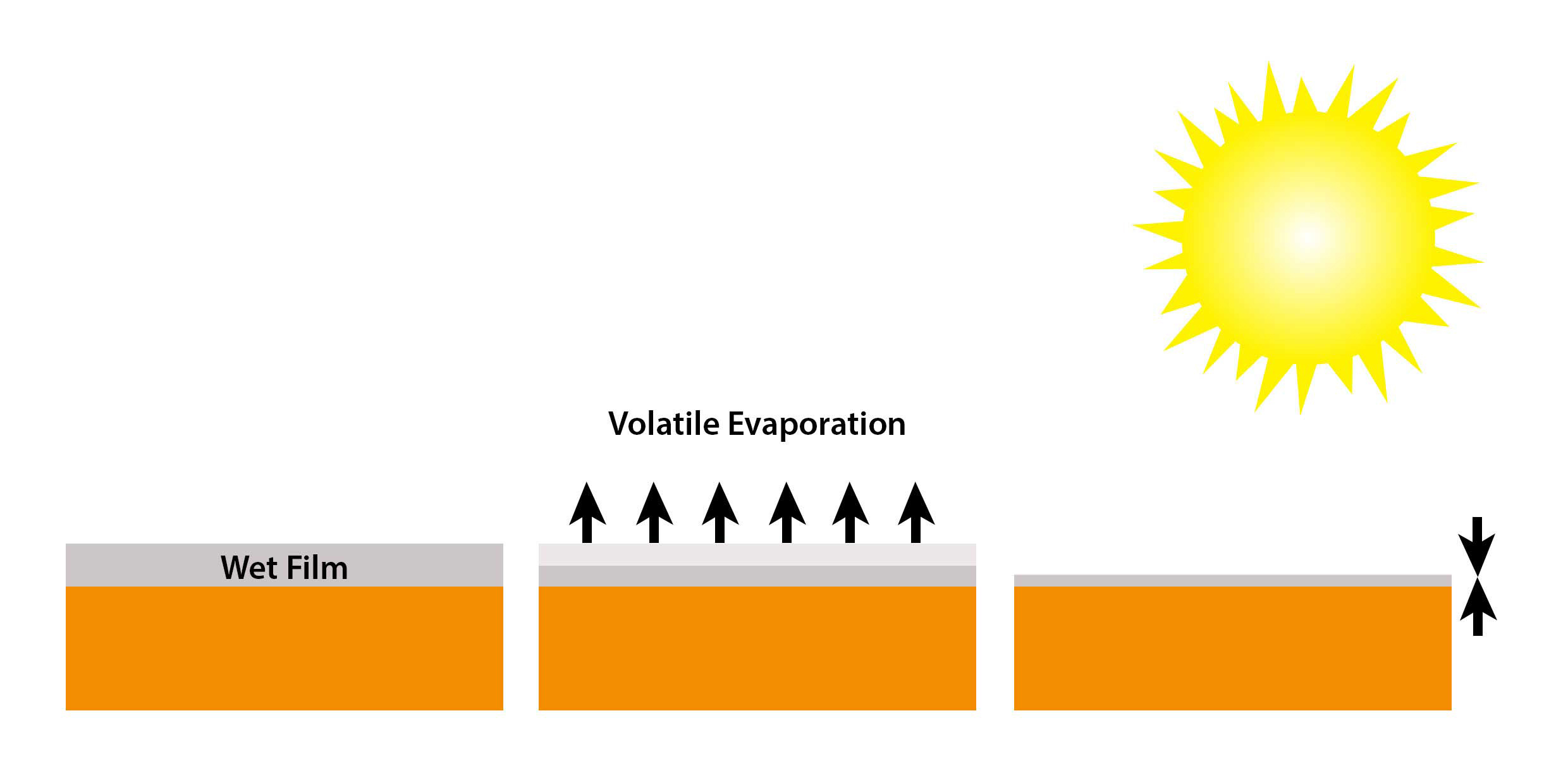## Calculating Dry Film Thickness

Paint consists of volatile and non-volatile components. When the paint is applied to a surface, the volatile components evaporate, leaving the non-volatile solids on the surface.

The ratio of volatile/non-volatile components, known as volume solids, is quoted in the technical data sheet of the coating concerned. The components are often written as a ratio or as a percentage and can be used to predict the dry film thickness when the wet film thickness is known.

The predicted dry film thickness (DFT) can be calculated from the wet film thickness (WFT) using the following formula:

## DFT = WFT x Volume SolidsFig 1. Evaporation of volatile components

## Example

Paint A has a volume solids of 50% and a wet film thickness measured at 250μm (10 mils)

DFT = 250 x 50% = 125μm (5 mils)

Paint B has a volume solids of 70% and the specification requires a dry film thickness of 100µm (4 mils)

WFT=DFT/Volume solids=100/0.7= 143µm (5.7 mils)

Paint C has a volume solids (wet to dry ratio) of 3:1 and a measured wet film thickness of 300μm (12 mils)

DFT = 300 x ⅓ = 100μm (4 mils)